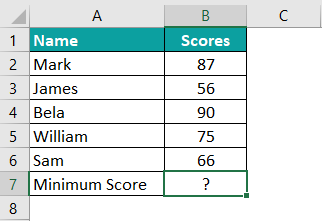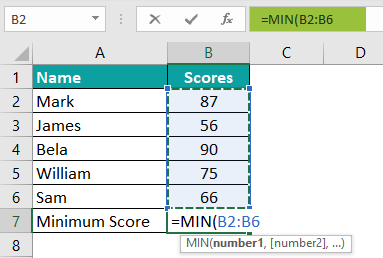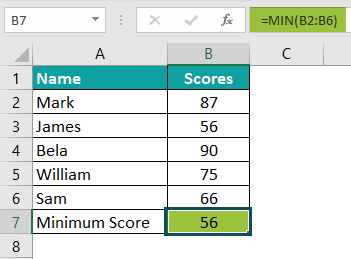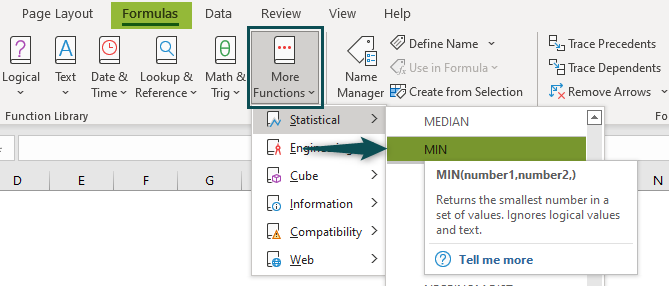# MIN Excel FunctionArticle byExcelMojo Team## MIN Function in Excel

The MIN function of Excel finds the minimum (or smallest) value from a list of numeric values. It is a Statistical function of Excel, which returns only a numeric value as the output. The MIN function is available in almost all versions of Excel.

For example, in the following image, the formula “=MIN(A2:A6)” helps find the minimum value from the numbers of column A.

The output of the MIN formula is 2.

###### Key Takeaways
• The MIN function of Excel helps find the minimum value from a list of numeric values. It is a Statistical function of Excel.
• The syntax of the MIN function is “MIN(number1,[number2],…).” The first number is mandatory, while the subsequent numbers are optional.
• The MIN function returns 0 when none of the arguments is numeric or a blank range has been supplied.
• The MINA function helps find the minimum value from a list of numbers, logical values (true and false), and text representations of numbers.

### MIN() Formula in Excel

The syntax of the MIN function of Excel is shown in the following image:

The MIN function accepts the following arguments:

• Number1: This is the first number, cell reference or range whose minimum needs to be found.
• Number2: This is the second number, cell reference or range whose minimum needs to be found.

The “number1” is a mandatory argument, while the subsequent number arguments are optional. The arguments can also be supplied as named ranges containing numbers.

The MIN function treats the empty cells, logical values (true and false), and text strings as follows:

• It ignores the cell references of empty cells, logical values, and text strings.
• It counts those logical values that are supplied to it directly. The values “true” and “false” are counted as 1 and 0 respectively.
• It counts the text representations of numbers irrespective of whether they are supplied directly (in double quotes) or as cell references. For instance, the formula =MIN(5,9,“4”) returns 4.
• It returns the “#VALUE!” error when text strings are supplied to it directly within double quotes. For instance, the formula =MIN(1,2,”t”,”r”) returns the “#VALUE!” error.

Note: The MIN function can accept up to 255 arguments in Excel 2007 and the newer versions.

### How to Use the MIN Function in Excel?

The MIN function of Excel can be used in the following ways:

#1 – Access the MIN Function from the Excel ribbon

1. Choose an empty cell that will contain the output.

2. Go to the Formulas tab of Excel.

3. Click the “more functions” drop-down from the “function library” group.

4. Select “statistical” and then choose “MIN” from the list of functions. A window called “function arguments” opens.

5. Enter the arguments in the “number1” and “number2” boxes.

6. Click “Ok” to find the minimum value.

#2 – Enter the MIN function in the worksheet manually

1. Select an empty cell for the output.
2. Type “=MIN(” in the selected cell. Alternatively, type “=M” and double-click the MIN function from the list of suggestions shown by Excel.
3. Enter the arguments as cell references or direct values.
4. Close the parenthesis and press the “Enter” key.

### Basic Example

The succeeding image shows the names of fruits and their prices (in \$) in columns A and B respectively. Find the fruit that has the minimum price. Use the MIN function of Excel.

The steps to find the minimum price are listed as follows:

Step 1:  Select an empty cell for the output. We have selected cell B7.

Step 2:  Enter the following MIN formula (without the double quotation marks) in cell B7.

“=MIN(B2:B6)”

For entering this formula, open the MIN function by typing “=MIN” followed by the opening parenthesis. Next, either select the range of cells beginning from B2 to B6 or type the range B2:B6 in the MIN formula manually.

Step 3:  Press the “Enter” key. The output in cell B7 is 40, as shown in the following image. So, banana has the least price compared to the other fruit prices.

#### Example #1–Using the MIN Function When the Inputs are in Different Formats

The succeeding image shows some numbers as integers, decimals, and percentages in column A. We want to perform the following tasks in Excel:

• Find the minimum value from the range A2:A7.
• Show whether or not the blank cell is included while looking for the minimum value in the stated range.

Use the MIN function of Excel.

The steps to perform the given tasks are listed as follows:

Step 1: Select cell B2 for the output.

Step 2: Enter the following MIN formula in cell B2. In this formula too, one can either select the numerical range of column A or type the references manually.

“=MIN(A2:A7)”

Step 3: Press the “Enter” key. The output in cell B2 is 5%, as shown in the following image.

Hence, the MIN function has returned the correct minimum value, which is 0.05. However, the function has not taken into account the blank cell. The MIN function has simply ignored the empty cell A6 while finding the minimum value.

#### MINA Function in Excel

The MINA and the MIN functions are of the same family. The former finds the minimum value from a list of numbers, logical values (true and false), and text representations of numbers.

The syntax of the MINA function of Excel is stated as follows:

“MINA(value1,[value2],…)”

The arguments can be numbers, text representations of numbers, logical values or cell references. The “value1” argument is required, while the subsequent value arguments are optional.

The MINA function of Excel treats the empty cells, logical values (true and false), and text strings as follows:

• It ignores the empty cells.
• It counts the logical values “true” and “false” as 1 and 0, irrespective of whether they are supplied directly or as cell references.
• It counts the cell references containing text strings as 0. However, if text strings are supplied directly to the function in double quotes, they result in the “#VALUE!” error. For instance, the formula =MINA(4,”b”,8,2) returns the “#VALUE!” error.
• It counts the text representation of numbers, whether supplied directly or as cell references. For instance, the formula =MINA(12,6,“2”) returns 2.

Note: The MINA function can accept up to 255 values in Excel 2007 and the newer versions.

#### Example #2–Using the MINA Function When the Inputs are Numeric, Textual, and Logical

The succeeding image shows some numeric, textual, and logical values. Find the minimum value from the range A2:A7. Use the MINA function of Excel.

The steps to find the minimum number in the stated range are listed as follows:

Step 1: Select cell B2. The output will be displayed in this cell.

Step 2: Enter the following formula in cell B2.

“=MINA(A2:A7)”

The range can be entered the way one did in step 2 of the basic example or example #1.

Step 3: Press the “Enter” key. The output in cell B2 is 0, as shown in the following image.

Therefore, the MINA function returns the minimum value from the list of numbers, text strings, and logical values. Since the function counts the text string (ABCD) and the logical value “false” as zeroes, the minimum value of the range A2:A7 is 0.

#### Example #3–Using the MIN Function When the Input is a Blank Cell Reference

The succeeding image shows cell A2 as a blank cell. Find the minimum value by supplying this single cell reference to the MIN function.

The steps to find the minimum value by supplying a blank cell are listed as follows:

Step 1: Select cell B2.

Step 2: Enter the following formula (without the double quotation marks) in cell B2.

“=MIN(A2)”

Step 3: Press the “Enter” key. The output is 0. This is shown in cell B2 of the following image.

Since the MIN function has been supplied a blank cell reference, it returns 0. However, had there been a range consisting of a mix of numeric values and empty cells, the MIN function would have ignored the empty cells. In this case, the MIN function would have returned the minimum of the numeric values.

Hence, one can say that the MIN function takes into account only the numeric cells while finding the minimum value.

#### Example #4–Using the MIN Function When the Minimum Value is to be Highlighted

The succeeding image shows some items and their sales revenue (in \$) in columns A and B respectively. Highlight the minimum revenue with the help of the MIN function.

The steps to highlight the minimum revenue by using the MIN function are listed as follows:

Step 1: Select the column within which the minimum value must be highlighted. We have selected column B.

Step 2: Perform the following actions in Excel:

• Click the “conditional formatting” drop-down from the “styles” group of the Home tab.
• Expand the option “top/bottom rules” and select “bottom 10 items.”

The selections are shown in the following image.

Step 3: A window called “bottom 10 items” opens, as shown in the following image. Perform the following actions in this window:

• In the box to the left, enter 1. This is because only one value, which is the minimum, needs to be highlighted.
• In the box to the right, select the desired color. We have chosen “light red fill with dark red text.”
• Click “OK.”

Step 4: The revenue generated by the strawberry shake (\$50) is highlighted in column B. This is the minimum revenue, as shown in the following image.

### Important Things to Note

The important points related to the MIN function of Excel are listed as follows:

• It considers only the numeric values for finding the minimum.
• It ignores the the cell references of blank cells, textual, and logical values. However, text strings supplied directly to the function (within double quotes) result in the “#VALUE!” error. But, the logical values supplied directly are counted by the function.
• It does not sum the numeric values.
• It can handle up to 255 arguments.

1. Define the MIN function of Excel.

The MIN function finds the minimum value from a series of numerical values. The function can also be supplied cell references, range references or named ranges containing numbers. It is an in-built function of Excel and is categorized as a Statistical function.

The syntax of the MIN function of Excel is stated as follows:

“MIN(number1,[number2],…)”

2. How to use the MIN function in Excel?

Let us consider an example to understand how to use the MIN function in Excel. The steps for using this function are listed as follows:

Step 1: Select an empty cell for the output, like cell B7 in this example.Step 2: Enter the following MIN formula in cell B7. Exclude the beginning and ending double quotation marks while entering this formula in Excel.
“=MIN(B2:B6)”Step 3: Press the “Enter” key. The output is 56, as shown in the following image.3. Where is the MIN function in Excel?

The steps to access the MIN function in Excel are listed as follows:

a. Click the “more functions” drop-down (in the “function library” group) from the Formulas tab of Excel.
b. Expand the option “statistical” and choose “MIN.”
c. Specify the arguments in the “function arguments” window.
d. Click “Ok” to find the minimum value.

The selections of steps “a” and “b” are shown in the following image.